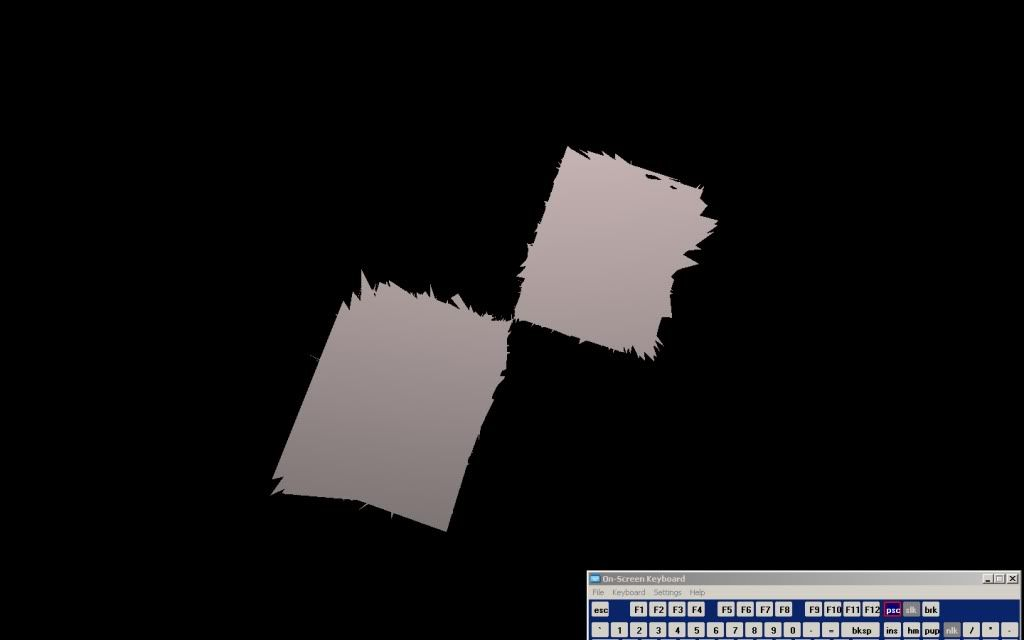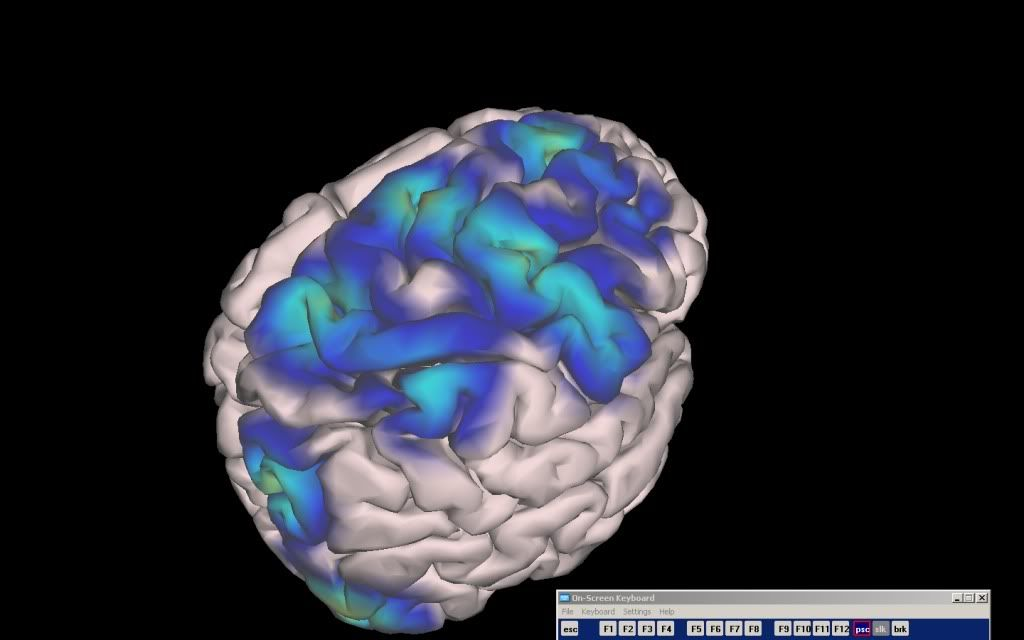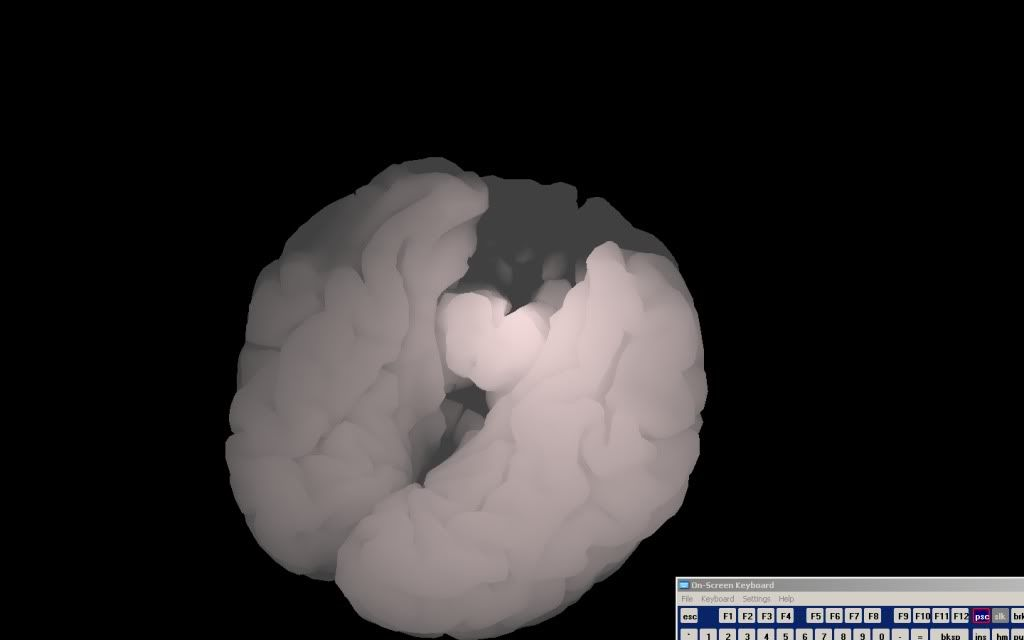# VBO and IBO

glEnableClientState(GL_VERTEX_ARRAY);

GLuint VertexVBOID;
glGenBuffers(1, VertexVBOID);
glBindBuffer(GL_ARRAY_BUFFER, VertexVBOID);
glBufferData(GL_ARRAY_BUFFER, ((VertexTotal*3) * sizeof(float)), electrodexyz, GL_STATIC_DRAW);

GLuint IndexVBOID;
glGenBuffers(1, &IndexVBOID);
glBindBuffer(GL_ELEMENT_ARRAY_BUFFER, IndexVBOID);
glBufferData(GL_ELEMENT_ARRAY_BUFFER, (VertexTotal) * sizeof(unsigned int), indices, GL_STATIC_DRAW);

glVertexPointer(3, GL_FLOAT, 0, 0);
glDrawElements(GL_TRIANGLES, (VertexTotal), GL_UNSIGNED_INT, 0);

I’m currently using this to render a model of 50,000 vertices. The problem is that when rendering I get this output, rather than a brain model. I believe the indices must be wrong, but I can’t determine how. When importing the model I add each x,y,z coord to an array of 3 times the number of vertices (for x,y,z).Then for each vertex I add count++ to the value of the indices, example array = 1; array = 2; array[n] = n+1;

Am I approaching using VBO’s and IBO’s wrong?

Help is greatly appreciated

glBufferData(GL_ELEMENT_ARRAY_BUFFER, (VertexTotal) * sizeof(unsigned int), indices, GL_STATIC_DRAW);

VertexTotal sounds wrong to me in this line. Shouldn’t that be something like “numFaces*3” or similar?

VertexTotal actually is computing the amount of polygons on the model. I mistakenly, originally, named it VertexTotal.

Should my bufferdata parameters of the VBO be different?

VertexTotal actually is computing the amount of polygons on the model. I mistakenly, originally, named it VertexTotal.

Should my bufferdata parameters of the VBO be different?

Please give correct names to your variable a post your code again if the same problem occurs so that it is clear to us :).

You have to give the buffer size in bytes to glBufferData and the number of indices to glDrawElements.

glEnableClientState(GL_VERTEX_ARRAY);

GLuint VertexVBOID;
glGenBuffers(1, VertexVBOID);
glBindBuffer(GL_ARRAY_BUFFER, VertexVBOID);
glBufferData(GL_ARRAY_BUFFER, ((TriangleTotal*3) * sizeof(float)), electrodexyz, GL_STATIC_DRAW);

GLuint IndexVBOID;
glGenBuffers(1, &IndexVBOID);
glBindBuffer(GL_ELEMENT_ARRAY_BUFFER, IndexVBOID);
glBufferData(GL_ELEMENT_ARRAY_BUFFER, (TriangleTotal) * sizeof(unsigned int), indices, GL_STATIC_DRAW);

glVertexPointer(3, GL_FLOAT, 0, 0);
glDrawElements(GL_TRIANGLES, (TriangleTotal), GL_UNSIGNED_INT, 0);

And to Dynamically Allocate the Size of the Arrays for Indices and the Vertices

electrodexyz = new float[TriangleTotal*3];
indices = new unsigned int[TriangleTotal];

I think I am allocate the memory correctly? I pass the amount of Triangles to the VBO multiplied by 3 to include every point. I then pass the amount of triangles to the index buffer so there is 1 index per triangle? Am I approaching this incorrectly??

glBufferData(GL_ARRAY_BUFFER, ((TriangleTotal*3) * sizeof(float)), electrodexyz, GL_STATIC_DRAW);

That won’t work. If this code should reserve space for 3 individual vertices (each 3 floats) per triangle, you’ll need
(TriangleTotal*3)3sizeof(float) bytes.

Am I approaching this incorrectly??

yes. This is wrong. You should have 3 indices per triangle instead of only one.

As far as I can guess, you seem to have 3 individual vertices per triangles anyway (no vertex sharing among triangles?!). In this case, using glDrawArrays seems more appropriate and you don’t need the indices at all.

glBufferData(GL_ARRAY_BUFFER, ((TriangleTotal*3) * sizeof(float)), electrodexyz, GL_STATIC_DRAW);

I think it should be: 3 * ((TriangleTotal*3) * sizeof(float))

since there are 3 vertices per triangles and 3 coordinates per vertex.

And:

glBufferData(GL_ELEMENT_ARRAY_BUFFER, (TriangleTotal) * sizeof(unsigned int), indices, GL_STATIC_DRAW);

[b]3 * /b * sizeof(unsigned int) since there are 3 indexes per triangle.

Anyway, something is weird in your vertex data creation. You should not have as many vertices as indices. In your code you do not separate the number of indices and the number of vertices.
The purpose of indexed buffers id to use less vertices in your BO by not duplicate them.

That won’t work. If this code should reserve space for 3 individual vertices (each 3 floats) per triangle, you’ll need
(TriangleTotal*3)3sizeof(float) bytes.

I go through my model import and make a count for each time a triangle is rendered. Hence the 50,000 TriangleTotal.

I then allocated space for each array, TriangleTotal*3, for the array of vertices. 50,000 Tri’s and 3 floats per Tri. Then I allocated TriangleTotal for the Indices, I thought one index per triangle.

Using that formula I attempted to allocated TriangleTotal*3 for the total vertices in the array of vertices? For the array I input array=x1,array=y1,array=z1; array=x2,array=array=y2,array=z2, etc…

yes. This is wrong. You should have 3 indices per triangle instead of only one.

Why is it I should have three indices per Triangle? Sorry if this is a simple question, I am completely new to VBO’s and IBO’s, though I have tried to read about them before posting.

As far as I can guess, you seem to have 3 individual vertices per triangles anyway (no vertex sharing among triangles?!). In this case, using glDrawArrays seems more appropriate and you don’t need the indices at all.

I assume there is vertex sharing. I am not certain since I import the coordinates from a ASCII file. It is a high-res 50,000 poly brain model, vertex sharing seems necessary. If I use glDrawArrays, how would this optimize the rendering and leave out the IBO’s?

dletozeun,

I changed the bufferdata to the suggested size. I now get a “unhandled win32 exception” “access violation reading location”. If my memory is going out of bounds, I really am at a loss for what I’m doing wrong here.

To create the indices array, for each triangle that is rendered, in this order I add 1 to the array of indices. So indices = 1; indices = 2, etc…
And the positions, pos = x1, pos = y1, pos = z1, etc…

Update

I believe the error is not passing in an Interleave array with the glNormalPointer call? Without the normals, I’m sure this would create a mess of a rendering.

2 Questions:

1. Do I use the same data array of vertices for the normals? And how would I go about rendering the normals using a VBO?

2. As previously suggested, is glDrawArrays() going to compromise rendering speed? If I don’t use IBO’s will my program suffer?

Thanks everyone, especially dletozeun and skynet

Hum, I think you do not understand the idea behing indexed buffers. Some interesting lecture:

It is d3d documentation but it is quite general:
http://msdn.microsoft.com/en-us/library/bb147325(VS.85).aspx

OpenGL wiki:

dletozeun,

Yeah, I was alittle bit in the dark on indexed buffers. They seem to make some more sense. Do you know if glDrawArrays will compromise much performance since I am not sure how I would determine on a 50,000 poly model which are shared vertices in order to store in a list of indices.

Status:
The Rendering of the brain works now, I added normals. Except it is not appearing correct. Could you take a look? First is the normal, correct rendering using glCallList.

Second is the VBO’s with Normals I am attempting to get working.Code:
glEnableClientState(GL_VERTEX_ARRAY);
glEnableClientState(GL_COLOR_ARRAY);
glEnableClientState(GL_NORMAL_ARRAY);

glGenBuffers(1, &VertexVBOID);
glBindBuffer(GL_ARRAY_BUFFER, VertexVBOID);
glBufferData(GL_ARRAY_BUFFER, (3*(TriangleTotal*3) * sizeof(float)), electrodexyz, GL_STATIC_DRAW);

glGenBuffers(1, &IndexVBOID);
glBindBuffer(GL_ELEMENT_ARRAY_BUFFER, IndexVBOID);
glBufferData(GL_ELEMENT_ARRAY_BUFFER, 3 * (TriangleTotal*3) * sizeof(float), indices, GL_STATIC_DRAW); // indices are the normals

glGenBuffers(1, &ColorVBOID);
glBindBuffer(GL_ELEMENT_ARRAY_BUFFER, ColorVBOID);
glBufferData(GL_ELEMENT_ARRAY_BUFFER, 3 * (TriangleTotal*3) * sizeof(float), colorvalues, GL_STATIC_DRAW); // indices are the normals

glVertexPointer(3, GL_FLOAT, sizeof(float)*3, 0);
glNormalPointer(GL_FLOAT, sizeof(float)*3, NULL); //Normal start position address
glColorPointer(3,GL_FLOAT,sizeof(float)*3,NULL);

glDrawArrays(GL_TRIANGLES,0,TriangleTotal*3);

UPDATE

I changed the ELEMENT_ARRAY_BUFFER parameter for the BindBuffer and BufferData but now it will only attempt to draw the last array? Example, if I set normals to ARRAY_BUFFER after I set the positions, it will render a small sphere of all normals. If I set the colors last, it will render no primitives.

Any Clues?

3 floats per Tri

That’s the problem. it’s 9 floats, not 3. Each vertex is a 3-dimensional value, thus it takes 3 floats. Each triangle requires 3 vertices. Therefore, each triangle requires 9 floats.

Why is it I should have three indices per Triangle?

You are drawing your model using GL_TRIANGLES. This works by taking each 3 vertices and making a triangle out of them. So vertices 0, 1, 2 become a triangle; 3, 4, 5 become a triangle; etc.

Alfonse,

Yeah, I appreciate the explanation. I don’t know why I got confused by this but a previous post had some links to VBO and IBO information. Do you have any idea on how to bind the data for Postion, Normals, and Color somehow so I can render all together with a glDrawArrays() call?

That is the problem I’m stuck with now and any ideas about that would help out immensely!

glEnableClientState(GL_VERTEX_ARRAY);
glEnableClientState(GL_COLOR_ARRAY);
glEnableClientState(GL_NORMAL_ARRAY);

glGenBuffers(1, &VertexVBOID);
glBindBuffer(GL_ARRAY_BUFFER, VertexVBOID);
glBufferData(GL_ARRAY_BUFFER, (3*(TriangleTotal) * sizeof(float)), electrodexyz, GL_STATIC_DRAW);

// TriangleTotal=Vertices

glVertexPointer(3, GL_FLOAT, sizeof(float)*3, 0);

glGenBuffers(1, &IndexVBOID);
glBindBuffer(GL_ARRAY_BUFFER, IndexVBOID);
glBufferData(GL_ARRAY_BUFFER, 3 * (TriangleTotal) * sizeof(float), indices, GL_STATIC_DRAW); // indices are the normals

glGenBuffers(1, &ColorVBOID);
glBindBuffer(GL_ELEMENT_ARRAY_BUFFER, ColorVBOID);
glBufferData(GL_ELEMENT_ARRAY_BUFFER, 3 * (TriangleTotal) * sizeof(float), colorvalues, GL_STATIC_DRAW);

glColorPointer(3,GL_FLOAT,sizeof(float)*3,0);
glDrawArrays(GL_TRIANGLES,0,(TriangleTotal
3));

By Placing the Vertex Pointer above the Buffer Data for color and normals AND by switching colorpointer with normalpointer, I get the perfectly rendered brain as shown in the above post?

Why would this be? And how could I go about adding a seperate Vertex Attribute Buffer that updates each frame?

VBO is designed specifically to work almost exactly like regular vertex arrays. So first, write the code as though you were not using buffer objects.

Do you know if glDrawArrays will compromise much performance since I am not sure how I would determine on a 50,000 poly model which are shared vertices in order to store in a list of indices.

I can’t say which of indexed or non indexed arrays are faster. I think it mostly depends on the vertex buffer usage.
When you reach a fair amount of vertex data, indexed buffers would certainly be faster since less data is involved… They are particularly efficient when vertex data often need to be updated. Furthermore, using indexed vertex buffers require much less memory space.
For static usage, simple non indexed vertex buffer may be suitable but I can’t say to what extent. So IMO, it is more about vertex buffer usage and memory occupation that performances. And mesh data exported from most of 3d modelers are stored in IBO styleBTW, how is build the brain mesh?

I am not sure to understand this post…
Does the code above works for you?

glVertexPointer(3, GL_FLOAT, sizeof(float)*3, 0);

Do you use interleaved arrays? I did not understand it before and I think it’s weird that you put a sizeof(float)*3 stride.

If your vertices are tightly packed in the VBO it should be zero.

BTW, how is build the brain mesh?

I am importing into openGL using MilkShape ASCII format since I have to get this model from MATLAB. I convert patches to STL and then import to Milkshape -> then to ASCII for my openGL program.

I am not sure to understand this post…
Does the code above works for you?

Apologies! I discovered my error. I was resetting the color in my fragment shader, removing the gl_Color call I had in my vertex shader. How would I go about adding a Vertex Attribute Buffer Object? That is the last thing I need to do and I’ll be done! So close I can taste it.

Do you use interleaved arrays? I did not understand it before and I think it’s weird that you put a sizeof(float)*3 stride.

If your vertices are tightly packed in the VBO it should be zero.

I do not use interleaved arrays. I made a mistake in the code. There should be zero stride because I merely use multiple buffers.
Thanks dletozeun

The same way as other vbo. I thought you already solved this problem, didn’t you?

Some code you posted:

``````
// position vertex attribute
glEnableVertexAttribArray(index);
glVertexAttribPointer(index, kVertices, GL_FLOAT, 0, BUFFER_OFFSET(0));

``````

Almost like you enabled vertex array client side capabilities for vertex position, color and normal attributes, you may enable generic vertex attributes calling glEnableVertexAttribArray with the location of your custom attribute in your vertex shader.

The same way as other vbo. I thought you already solved this problem, didn’t you?

Well, I should had stated more clearly. I have a vertex attribute array being used right now. Thanks to that code. But I couldn’t determine if I was implementing something incorrectly since my CPU is going up to 50% from 5% by the

glBufferData(GL_ARRAY_BUFFER, (TriangleTotal) * sizeof(float), activationArrayTotal, GL_DYNAMIC_DRAW);

The Total number of vertices is 150,000 (50,000 tri’s * 3); Triangle Total represents that 150,000. If I am updating a buffer of 150,000 elements each frame should my CPU really get hit with 50% usage?

If you can recommend any optimizations or perhaps I’m doing something incredibly wrong! Thanks